International
Tables for
Crystallography
Volume B
Reciprocal space
Edited by U. Shmueli

International Tables for Crystallography (2006). Vol. B. ch. 2.1, pp. 195-196   | 1 | 2 |

## Section 2.1.5.1. Ideal acentric distributions

U. Shmuelia* and A. J. C. Wilsonb

aSchool of Chemistry, Tel Aviv University, Tel Aviv 69 978, Israel, and bSt John's College, Cambridge, England
Correspondence e-mail:  ushmueli@post.tau.ac.il

#### 2.1.5.1. Ideal acentric distributions

| top | pdf |

The ideal acentric distributions are obtained by applying the central-limit theorem to the real and the imaginary parts of the structure factor, as given by equation (2.1.1.1). Consider first a crystal with no rotational symmetry (space group P1). The real part, A, of the structure factor is then given by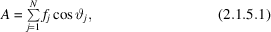where N is the number of atoms in the unit cell and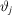is the phase angle of the jth atom. The central-limit theorem then states that A tends to be normally distributed about its mean value with variance equal to its mean-square deviation from its mean. Under the assumption that the phase anglesare uniformly distributed on the 0–2π range, the mean value of each cosine is zero, so that its variance is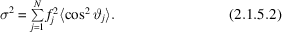Under the same assumption, the mean value of each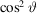is one-half, so that the variance becomes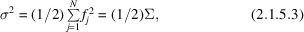where Σ is the sum of the squares of the atomic scattering factors [cf. equation (2.1.2.4)]. The asymptotic form of the distribution of A is therefore given by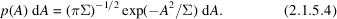A similar calculation, with sines instead of cosines, gives an analogous distribution for the imaginary part B, so that the joint probability of the real and imaginary parts of F is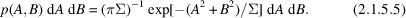Ordinarily, however, we are more interested in the distribution of the magnitude,, of the structure factor than in the distribution of A and B. Using polar coordinates in equation (2.1.5.5)[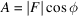,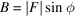] and integrating over the angle φ gives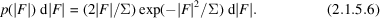It is usually convenient, in structure-factor and intensity statistics, to express the results in terms of the normalized structure factor E and its magnitude. Ifhas been put on an absolute scale (see Section 2.2.4.3), we have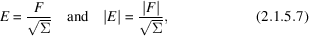so that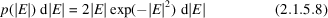is the normalized-structure-factor version of (2.1.5.6).

Distributions resulting from noncentrosymmetric crystals are known as acentric distributions; those arising from centrosymmetric crystals are known as centric. These adjectives are used to describe distributions, not crystal symmetry.